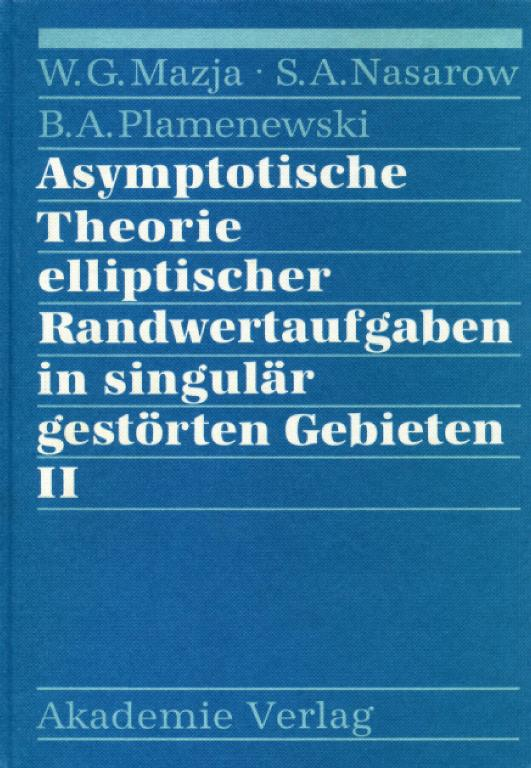# Asymptotische Theorie elliptischer Randwertaufgaben in singulär gestörten Gebieten II

Mazja, W. G.; Nasarow, S. A.; Plamenewski, B. A.,

The authors continue their investigations from Volume I [MR 92g:35059], employing the same general principles. Further, they consider elliptic boundary value problems the solutions of which are singularly perturbed caused by several degenerations of the domain. This concerns, especially, the following ones: (1) domains with narrow cavities, e.g., with tubes, notches, or cracks removed; (2) domains consisting of some parts which are connected merely by narrow passages; (3) narrow domains, i.e., the expansions in some directions are very small compared with the other ones (including, e.g., domains with closely neighbouring parallel cracks). Here "small" or "narrow" is measured by a positive parameteron which the domain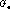in question depends; for,will degenerate: e.g.,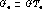with a "normal" domain G in, and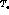a torus of thickness, will tend to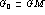where M is a closed curve in G.

The main subject is the construction of asymptotic expansions, for, of the solutions of the problems posed in. This is done, as in Volume I, by iteration processes which consist of solving, in alternating fashion, certain limit problems (two, in general) where at least one of them depends on parameters: one problem in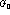, and another one, e.g., in the complement of the model of the cavity (hole) removed from G. As usual in singular perturbation problems, boundary layers may arise.

The proofs of solvability of the limit problems, and of the asymptotic expansions of the solutions, rely essentially on results of the modern theory of elliptic boundary value problems in piecewise smoothly bounded domains. The constructions of the expansions here are mostly far more complicated then in the cases of singular perturbations of local type considered in Volume I. Fortunately, most problems are first presented in a simpler model setting (e.g., Poisson's equation in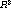).

In the last part (Chapter VII) of the book, asymptotic expansions of solutions are, by similiar methods, constructed for elliptic boundary value problems with rapidly periodically oscillating coefficients, but also for differential or difference equations on-periodic curvilinear nets in; especially, the corresponding homogenized differential operators are derived.

Moreover, an interesting analysis of the paradoxa is given that may occur with the solution of plane boundary value problems if a smooth boundary is approximated by polygons.

Reviewed by Dietrich Gohde